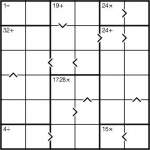Inequality Calcudoku puzzle

Here’s a fun little puzzle – a Calcudoku Inequality puzzle.

Just place 1 to 6 into each row and column whilst obeying the bold-lined cages’ operator totals. These give the result of applying the stated operator between all numbers in that region, so for example the result of adding together all the squares in a ‘24+’ region must be 24. Similarly the squares in the 1728x region must all multiply to that total. Unlike in Killer Sudoku, you can repeat a value within a cage (but you must still obey the constraint to not repeat a number in a row or column).

There are also some inequalities marked. These show that the value of one square is lower than the value of a square next to it. The arrow always points to the smaller number.

Good luck!